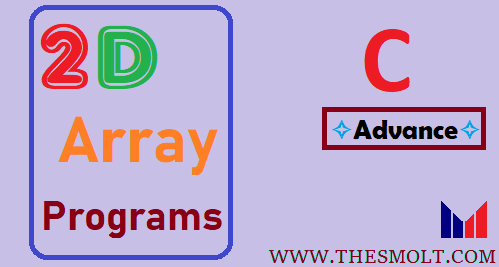# 2d array programs in C

In this program, we learn 2d array programs in C, and How to pass 2D array in Function and Passing Array with 2d array programs in C with Pointer Help of two-dimensional array.

## How to pass 2d array in function

```#include <stdio.h>

#define M 3
#define N 3

void print(int arr[M][N])
{
int i, j;
for (i = 0; i < M; i++){
for (j = 0; j < N; j++)
printf("%d ", arr[i][j]);
printf("\n");
}
}

int main()
{
int arr[] = {{1, 2, 3}, {4, 5, 6}, {7, 8, 9}};
print(arr);
return 0;
}```
Output
1 2 3

4 5 6

7 8 9

You can also try one way is this, where the index of both dimensions are known, like in this case M and N which are 3

## Pass 2d array as parameter in C

If you don’t understand the first code, then you can try this, this is more clearly then 1st..tRy this

```#include <stdio.h>

#define M 3
#define N 3

void print(int arr[M][N])
{
int i, j;
for (i = 0; i < M; i++){
for (j = 0; j < N; j++)
printf("%d ", arr[i][j]);
printf("\n");
}
}

int main()
{
int arr[M][N] = {{1, 2, 3}, {4, 5, 6}, {7, 8, 9}};
print(arr);
return 0;
}```
Output
1 2 3

4 5 6

7 8 9

#### Find sum of Matrix using Array (click)

or void print(int arr[][N], int firstDim); here the size of the dim is already known like, #define N 3, but the size of the firstDim i will be passed as a parameter when we call the print function in main, like print(arr,3);  We can pass it using a pointer like this.

## 2d array using pointers

```#include <stdio.h>
void print(int *arr, int m, int n)
{
int i, j;
for (i = 0; i < m; i++)
for (j = 0; j < n; j++)
printf("%d ", *((arr+i*n) + j));
}

int main()
{
int arr[] = {{1, 2, 3}, {4, 5, 6}, {7, 8, 9}};
int m = 3, n = 3;

// We can also use "print(&arr, m, n);"
print((int *)arr, m, n);
return 0;
}```
Output
1 2 3

4 5 6

7 8 9## How to declare 2d array in function

About the declaration of two dimensional Array in the c Program, that we learn it via using 3 different Programs.

END

This site uses Akismet to reduce spam. Learn how your comment data is processed.